# 基于文丘里混合的多孔介质燃烧火焰数值模拟研究Numerical Simulation Study of Incompletely Mixed Gas in Porous Media Combustion Flame Based on Venturi Mixture

DOI: 10.12677/MOS.2021.101005, PDF, HTML, XML, 下载: 89  浏览: 174  科研立项经费支持

Abstract: The fuel/air Venturi mixer is widely used in the industrial premixed combustion. The uniformity of the mixed gas has a great influence on the combustion performance. In order to study the com-bustion characteristics of non-uniform mixed gas in porous media, this paper studied the me-thane/air non-uniform premixed gas after Venturi mixing and the Spatial Mixing Deficiency (SMD) is about 2%, when entering in the double-layer porous media burner. The research results show that: due to the influence of uneven mixing, non-premixed gas easily leads to local high tempera-ture when ignited and burned in the porous medium and reduces the service life of the burner. When stable combustion is achieved, due to the good thermal conductivity of porous medium, the local high temperature can be flattened, but flame stability becomes worse.

1. 引言

2. 数值模拟

2.1. 物理模型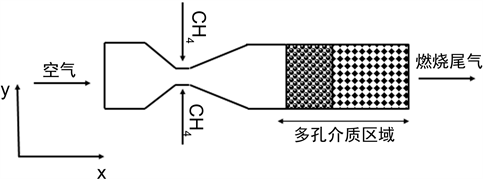Figure 1. Schematic diagram of porous burnerTable 1. Thermo-physical properties of Al2O3 and SiC

2.2. 计算方法

1) 假设气体为不可压缩流体；

2) 多孔介质骨架为灰体且各向同性；

3) 多孔介质对燃烧反应没有催化作用并忽略由于多孔介质对气体的阻挡而产生的扰动对甲烷和空气产生的混和，既忽略弥散效应；

4) 忽略重力对气体的作用；

5) 参与燃烧反应的各组分以及燃烧产物为不可压缩理想气体。

6) 气体混合物在燃烧过程中各组分的化学性质(活化能Ea，指前因子等)不变。

1) 连续性方程

$\frac{\partial \left(\epsilon {\rho }_{g}\right)}{\partial t}+\nabla \cdot \left(\epsilon {\rho }_{g}v\right)=0$ (1)

2) 动量守恒方程

$\frac{\partial \left(\epsilon {\rho }_{g}vv\right)}{\partial t}+\nabla \cdot \left(\epsilon {\rho }_{g}vv\right)=-\nabla p+\nabla \cdot \left(\mu \nabla v\right)+f-{R}_{p}$ (2)

${R}_{p}=\frac{\mu }{\alpha }v+\frac{{C}_{2}{\rho }_{g}}{2}|v|v$ (3)

${R}_{p}=\frac{A\mu {\left(1-\epsilon \right)}^{2}}{{D}_{p}^{2}{\epsilon }^{3}}v+\frac{2B\left(1-\epsilon \right)}{{D}_{p}^{}{\epsilon }^{3}}{\rho }_{g}|v|v$ (4)

(1) 组分输运方程

$\frac{\partial \left(\epsilon {\rho }_{g}{Y}_{i}\right)}{\partial t}+\nabla \cdot \left(\epsilon {\rho }_{g}v{Y}_{i}\right)=\nabla \cdot \left(\epsilon {\rho }_{g}{V}_{i}{Y}_{i}\right)+\epsilon \stackrel{˙}{\omega }{W}_{i}$ (5)

3) 能量方程

$\begin{array}{l}\frac{\partial \left({\left(\epsilon \rho {c}_{p}\right)}_{g}+{\left(\left(1-\epsilon \right)\rho {c}_{p}\right)}_{s}\right)T}{\partial t}+{\left(\rho {c}_{p}\right)}_{g}\nabla \cdot \left(\epsilon vT\right)+{\left(\rho {c}_{p}\right)}_{s}\nabla \cdot \left[\left(1-\epsilon \right)vT\right]\\ =\nabla \cdot \left({\lambda }_{eff}\nabla T\right)+\epsilon {\sum }_{i=1}^{i}{\stackrel{˙}{\omega }}_{i}{M}_{i}{h}_{i}\end{array}$ (6)

4) 理想气体状态方程

$pV=nRT$ (7)

2.3. 数值方法

2.4. 参数计算

${\text{CH}}_{\text{4}}+2L{\text{O}}_{\text{2}}+7.52L{\text{N}}_{\text{2}}={\text{CO}}_{\text{2}}+{\text{2H}}_{\text{2}}\text{O}+\left(2L-2\right){\text{O}}_{\text{2}}+7.52L{\text{N}}_{\text{2}}$ (8)

$\eta =\frac{1}{1+9.52\ast L}$ (9)

${v}_{ch4}=\frac{{V}_{in}}{{A}_{ch4}}=\frac{vA\eta }{{A}_{ch4}}$ (10)

${v}_{air}=\frac{{V}_{in}}{{A}_{air}}=\frac{vA\eta }{{A}_{air}}$ (11)

$\text{SMD}=\frac{\iint |c-\stackrel{¯}{c}|\text{d}A}{\stackrel{¯}{c}A}×100%$ (12)

2.5. 边界条件

${\lambda }_{eff}\frac{\partial {T}_{s}}{\partial x}=-\epsilon \sigma \left({T}^{4}-{T}_{0}^{4}\right)$

3. 计算结果及分析

3.1. 文丘里混合性能分析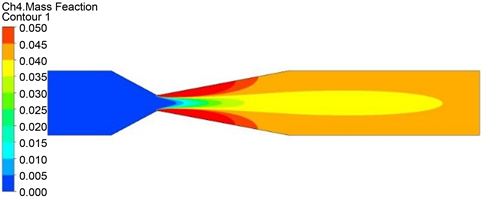Figure 2. Contour of methane mass fraction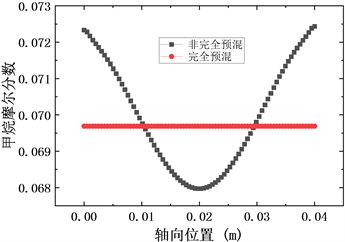Figure 3. Horizontal position distribution of methane mole fraction

3.2. 非均匀预混多孔介质燃烧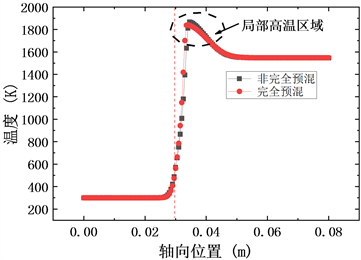(a) 3.6秒时两种模型轴向温度分布(b) 7.2秒时两种模型轴向温度分布(c) 18秒时两种模型轴向温度分布

Figure 4. Axial temperature distribution of the two models at different seconds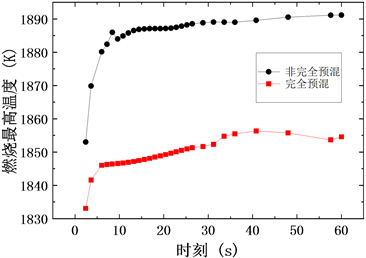Figure 5. Maximum combustion temperature at different times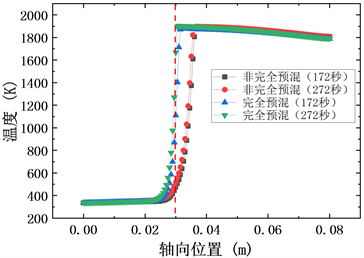Figure 6. Axial temperature distribution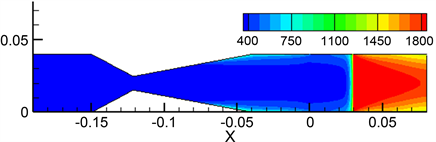(a) 完全预混燃烧(b) 非完全预混燃烧

Figure 7. Temperature contour of two combustion models (K)

4. 结论

1) 受到甲烷混合不均匀性的影响，非完全预混气体在多孔介质燃烧器中从点火开始到稳定燃烧的过程中容易产生局部高温而降低燃烧器的使用寿命。

2) 在达到稳定燃烧时，完全预混气体可以在多孔介质燃烧器内稳定燃烧，而预混不均匀使得非完全预混气体在多孔介质内的燃烧稳定性变差，其燃烧火焰发生了明显倾斜和弯曲。

NOTES

*通讯作者。

  程乐鸣, 岑可法, 周昊, 等. 多孔介质燃烧理论与技术[M]. 北京: 化学工业出版社, 2012.  Filho, R.C.M. and Pimenta, A.P. (2011) A Two-Dimensional Numerical Simulation of Combustion and Heat Transfer in Radiant Porous Burners. Combustion Science & Technology, 183, 370-389. https://doi.org/10.1080/00102202.2010.520278  Shi, J.R., Yu, C.M., Li, B.W., et al. (2013) Experimental and Numerical Studies on the Flame Instabilities in Porous Media. Fuel, 106, 674-681. https://doi.org/10.1016/j.fuel.2013.01.010  Hashemi, S.M. and Hashemi, S.A. (2017) Flame Stability Analysis of the Premixed Methane-Air Combustion in a Two-Layer Porous Media Burner by Numerical Simulation. Fuel, 202, 56-65. https://doi.org/10.1016/j.fuel.2017.04.008  Liu, H., Dong, S., Li, B.W., et al. (2010) Parametric Investigations of Premixed Methane-Air Combustion in Two-Section Porous Media by Numerical Simulation. Fuel, 89, 1736-1742. https://doi.org/10.1016/j.fuel.2009.06.001  Bubnovich, V., Henríquez, L. and Gnesdilov, N. (2007) Numerical Study of the Effect of the Diameter of Alumina Balls on Flame Stabilization in a Porous-Medium Burner. Numerical Heat Transfer, 52, 21. https://doi.org/10.1080/00397910601149942  Nemoda, S., Trimis, D. and Živković, G. (2004) Numerical Sim-ulation of Porous Burners and Hole Plate Surface Burners. Thermal Science, 8, 3-17. https://doi.org/10.2298/TSCI0401003N  Brenner, G., Pickenäcker, K, Pickenäcker, O., et al. (2000) Numerical and Experimental Investigation of Matrix-Stabilized Methane/Air Combustion in Porous Inert Media. Combustion and Flame, 123, 201-213. https://doi.org/10.1016/S0010-2180(00)00163-2  Barra, A.J., Diepvens, G., Ellzey, J.L., et al. (2003) Nu-merical Study of the Effects of Material Properties on Flame Stabilization in a Porous Burner. Combustion and Flame, 134, 369-379. https://doi.org/10.1016/S0010-2180(03)00125-1  郑成航, 程乐鸣, 李涛, 等. 多孔介质燃烧火焰面特性数值模拟[J]. 中国电机工程学报, 2009, 29(5): 48-53.  邵卫卫, 赵岩, 刘艳, 等. 燃气轮机燃烧室预混燃烧器天然气燃料/空气掺混均匀性研究[J]. 中国电机工程学报, 2017(3): 148-156.  李宇红, 孙宝成, 祁海鹰. 燃烧室预混段燃料/空气混合规律的数值研究[J]. 热能动力工程, 2008, 23(3): 235-239.  谢刚, 李宇红, 祁海鹰. DLN燃烧室的燃料-空气预混均匀性研究[J]. 工程热物理学报, 2006, 27(z2): 227-230.  邓彩华, 童亮, 陈壁峰, 等. 多孔介质流动的直接数值模拟[J]. 武汉理工大学学报: 交通科学与工程版, 2011, 35(6): 1257-1260.